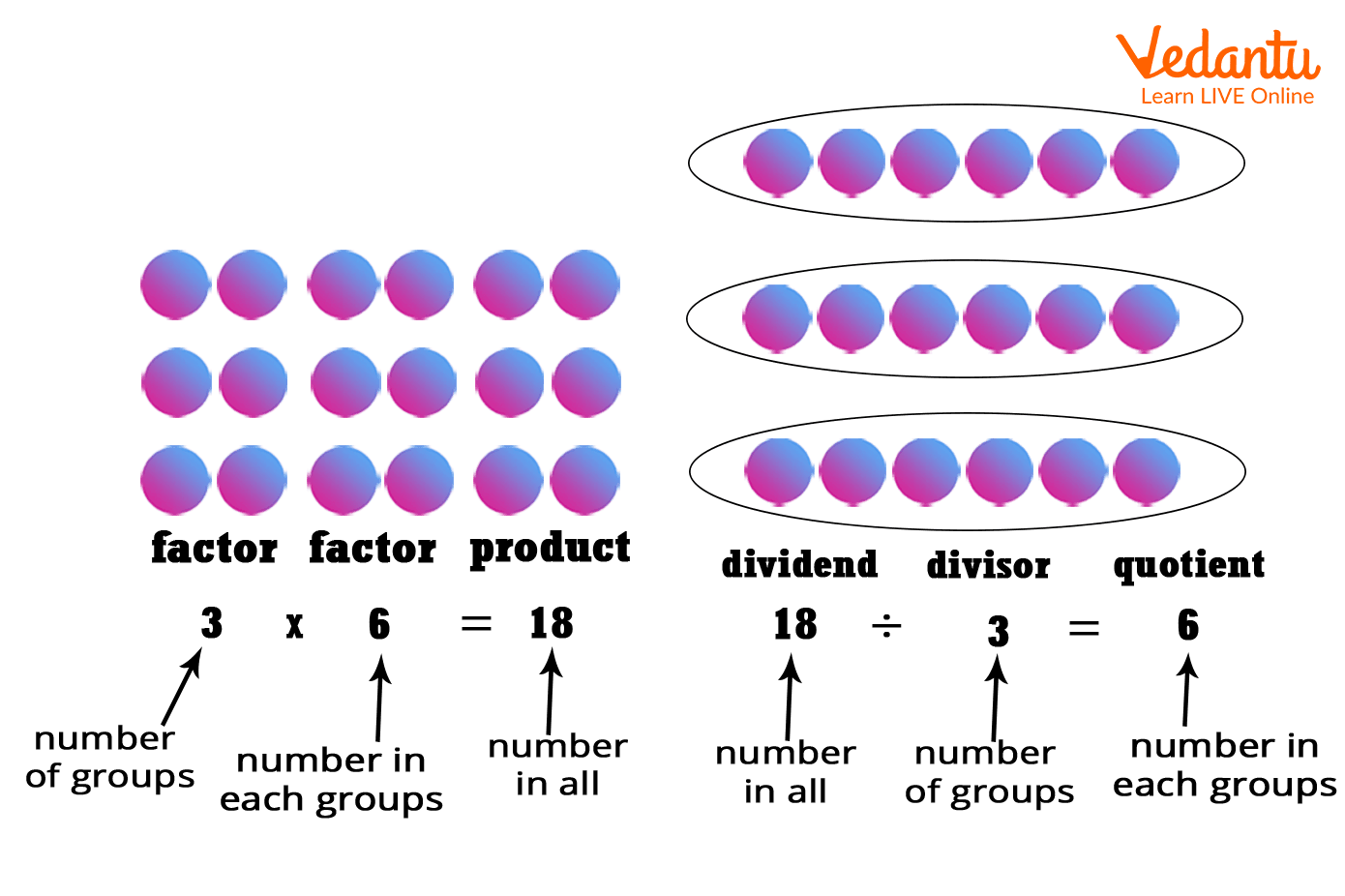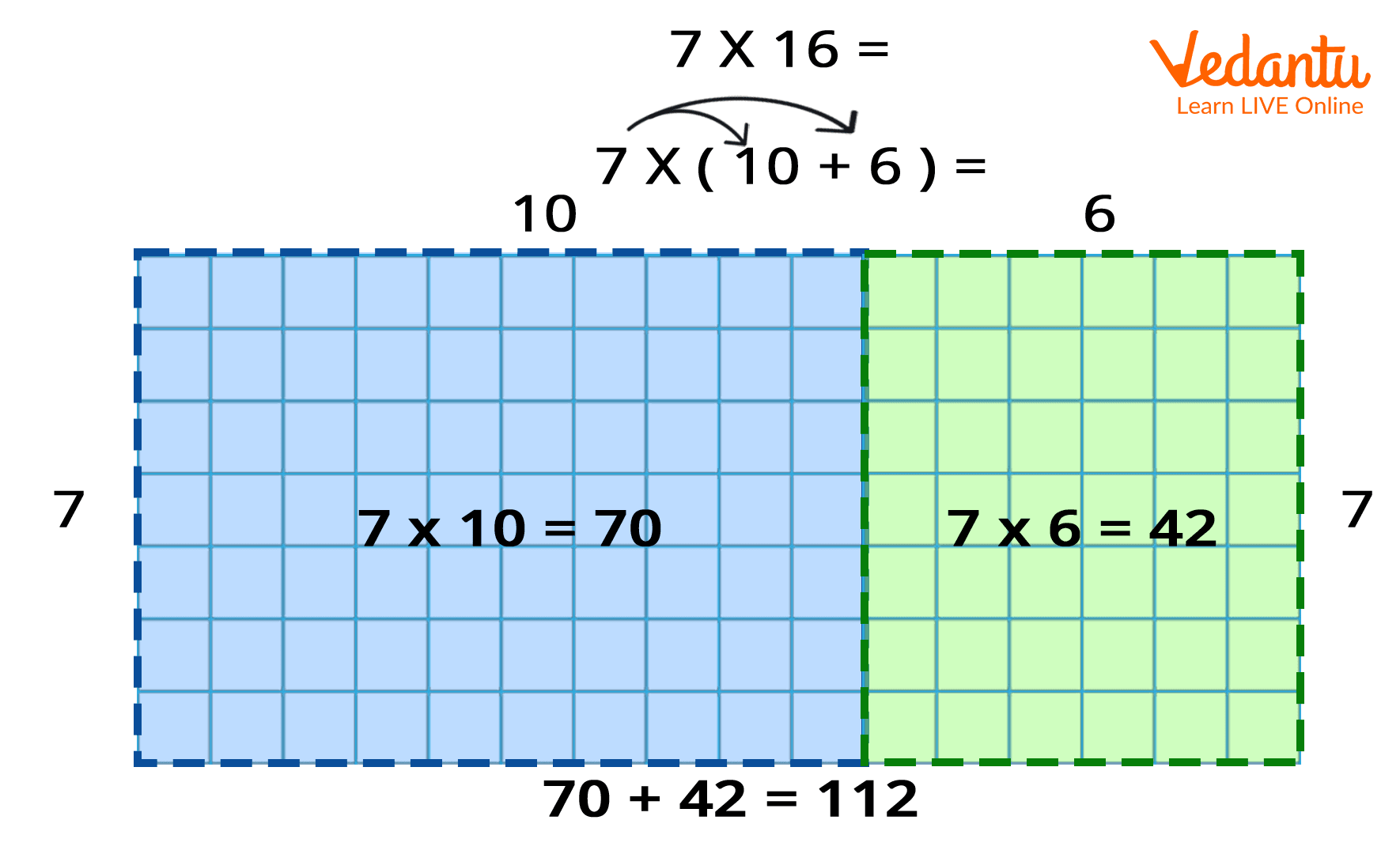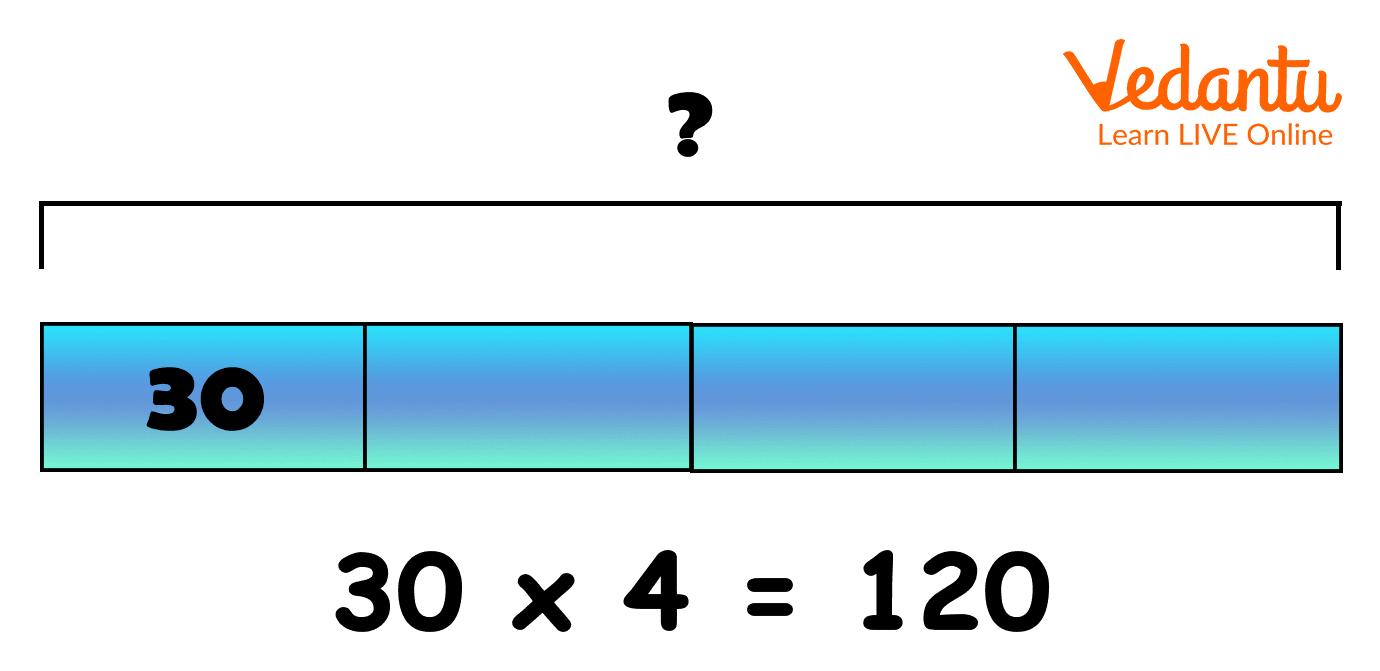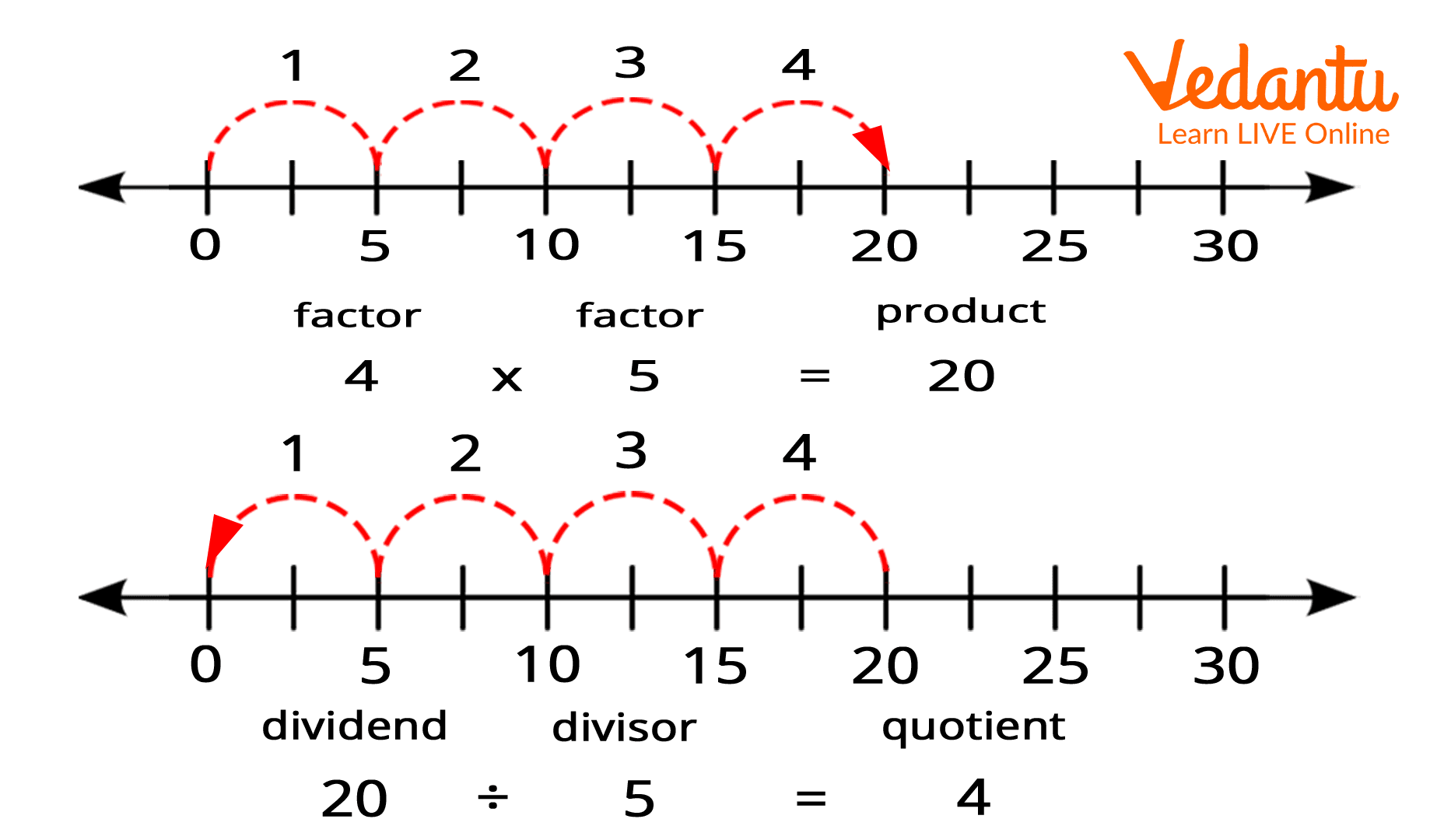Courses
Courses for Kids
Free study material
Free LIVE classes
More

# Multiplication and Division ModelsLIVE
Join Vedantu’s FREE Mastercalss

## Learn the Relation between Multiplication and Division

Students should have a clear understanding of Mathematical operations and not just memorise steps and procedures. For that, it is essential that students are able to analyse numbers and think about their relations. The algorithms of traditional multiplication and division are important to learn. To gain the full concept of this, we should start with a concrete concept and then move towards the pictorial and finally end with the abstract which will help students develop full mastery. In this article, there are some models that will be discussed which will help students to understand the relationship between multiplication and division.

## Examples of Multiplication and Division Models

The multiplication and division models are discussed below with examples.

1. Arrays

Array Model is one of the earliest models which is helpful in understanding the concept of multiplication and division. In this model, we can observe the connection between the two operations and can visually see the concept of “grouping” or “sharing”. An example of multiplication of 3 and 6 and division of 18 by 3 is given in the array model.Image: Example of arrays model of multiplication and division

1. Area Models

The area model is closely related to the computation with the standard algorithm. In this model, the difference is the visual representation and connection to the Base 10 System, and the understanding of place value. We can visually see the actual size of each computation and learn how to interpret the partial products. An example of multiplication of 7 and 16 is given below through the area model.Image: Example of area model

1. Bar Models

This model is based on the concept of equal groups and part-part-wholes. This model is helpful in moving away from the concrete phase and makes us familiar with the pictorial stage. Bar models are a good method for the students to show their thinking when problem-solving, especially when solving two-step problems. An example of multiplication of 30 and 4 is given below with the help of the bar model.Image: An example of the bar model of multiplication

1. Number Lines

Number lines help students to know the abstract stage of multiplication and division. They are allowed here to “skip” forwards or backwards direction distances to represent the inverse operation. Number lines are a traditional model which is taught to students to develop their thinking and explain the reasoning of multiplication and division. An example of multiplication of 4 and 5 and division of 20 by 5 is given below with the help of a number line.Image: Example of multiplication and division through number lines

## Conclusion

It is very important for the students to understand the actual meaning of the Mathematical operation. Just following the procedure of performing these operations is not enough. Hence, the models that describe the Mathematical operation are essential. In this article, we have discussed multiplication and division models with examples.

Last updated date: 22nd Sep 2023
Total views: 136.8k
Views today: 4.36k

## FAQs on Multiplication and Division Models

1. What are the models for multiplication and division?

The models for multiplication are the methods where the concept of multiplication and division is clearly visualised in some sort of way. These models help students to understand the concept of Mathematical operations when they are in the initial phase of learning Mathematical operations.

2. How do you model multiplication and division?

There are different models for multiplication and division such as array model, area model, bar model, and number lines. In these models, factors are represented by some visualisation which helps students to understand the process of multiplication and division.